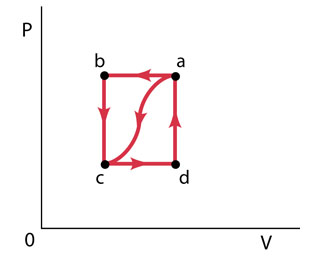Ask question

# Check (2 1,0 0),(0 0,2 0),(3 -1,0 0),(0 3, 0 1) is a bais for M22 or not?# Check (2 1,0 0),(0 0,2 0),(3 -1,0 0),(0 3, 0 1) is a bais for M22 or not?

Question
Matrix transformationsasked 2020-12-21
Check $$(2 1,0 0),(0 0,2 0),(3 -1,0 0),(0 3, 0 1)$$ is a bais for M22 or not?

## Answers (1)2020-12-22
Recall: Basis Let V be a vector space over a field F. A set of S of vectors in V is snid to be a basis of V if (i) S is linearly independent in V, and
(ii) S generates V ie. linear span of $$S, L(S) = V$$
Theorem: Let V be a vector space of dimension n over a field F. Then any linearly independent set of n vectors of V is a basis of V.
$$M_{22}$$ is the vector space of all $$2 \times 2$$ real matrices.
Let $$S = \left\{\left(\begin{array}{c}21\\ 00\end{array}\right), \left(\begin{array}{c}00\\ 21\end{array}\right), \left(\begin{array}{c}3-1\\ 00\end{array}\right),\left(\begin{array}{c}00\\ 31\end{array}\right)\right\}$$
We have to show that 5 is a basis of $$M_{22}$$
Let $$\alpha_{1} = \left\{\left(\begin{array}{c}21\\ 00\end{array}\right),\alpha_{2}= \left(\begin{array}{c}00\\ 21\end{array}\right),\alpha_{3}= \left(\begin{array}{c}3-1\\ 00\end{array}\right),\alpha_{4}=\left(\begin{array}{c}00\\ 31\end{array}\right)\right\}$$
Let us consider the relation
$$c_{1} \alpha_{1} + c_{2} \alpha_{2} + c_{3} \alpha_{3} + c_{4} \alpha_{4} = 0$$
where $$c_{1}, c_{2}, c_{3}, c_{4} \in RR.$$
Then we have $$c_{1} = \left(\begin{array}{c}21\\ 00\end{array}\right),+c_{2} \left(\begin{array}{c}00\\ 21\end{array}\right),+c_{3} \left(\begin{array}{c}3-1\\ 00\end{array}\right),+c_{4}\left(\begin{array}{c}00\\ 31\end{array}\right)=\left(\begin{array}{c}00\\ 00\end{array}\right) \Rightarrow \left(\begin{array}{c}2c_{1}+3c_{3}c_{1}-c_{3}\\ 2c_{2}+3c_{3}c_{2}+c_{4}\end{array}\right)=\left(\begin{array}{c}00\\00\end{array}\right)$$
Fron the above equation we get,
$$2c_{1} + 3c_{3} = 0$$
$$c_{1} - c_{3} = 0$$
$$2c_{2} + 3c_{4} = 0$$
$$c_{2} + c_{4} = 0$$
Solving these equation we get, $$c_{1} = 0, c_{2} = 0, c_{3} = 0, c_{4} = 0$$
This proves that the set $${\alpha_{1}, \alpha_{2}, \alpha_{3}, \alpha_{4}}$$ is lineary independent.
Now, we know that $$M_{22}$$ is a vector space of dimension 4 and its standard
basis is \left\{\left(\begin{array}{c}10\\00\end{array}\right),\left(\begin{array}{c}01\\ 00\end{array}\right), \left(\begin{array}{c}00\\ 10\end{array}\right), \left(\begin{array}{c}00\\ 01\end{array}\right)\right\}\)
As the dimension of $$M_{22}\ is\ 4\ and\ {\alpha_{1}, \alpha_{2}, \alpha_{2}, \alpha_{4}}$$ is a linearly independent
set containing 4 vectors of $$M_{22},$$
therefore the set $${\alpha_{1}, \alpha_{2}, \alpha_{3}, \alpha_{4}}$$
is a basis of $$M_22$$.
Result:
$$\left\{\left(\begin{array}{c}21\\00\end{array}\right),\left(\begin{array}{c}00\\ 21\end{array}\right), \left(\begin{array}{c}3-1\\ 00\end{array}\right), \left(\begin{array}{c}00\\ 31\end{array}\right)\right\}$$
is a basis for $$M_{22}$$

### Relevant Questionsasked 2021-01-24
It can be shown that the algebraic multiplicity of an eigenvalue lambda is always greater than or equal to the dimension of the eigenspace corresponding to lambda. Find h in the matrix A below such that the eigenspace for lambda = 5 is two-dimensional: $$\displaystyle{A}={\left[\begin{array}{cccc} {5}&-{2}&{6}&-{1}\\{0}&{3}&{h}&{0}\\{0}&{0}&{5}&{4}\\{0}&{0}&{0}&{1}\end{array}\right]}$$asked 2021-02-06
1:Find the determinant of the following mattrix $$[((2,-1,-6)),((-3,0,5)),((4,3,0))]$$ 2: If told that matrix A is singular Matrix find the possible value(s) for x $$A = { (16x, 4x),(x,9):}$$asked 2020-12-15
Find the values of k for which A is not invertible. Enter all values exactly in fractional form.
A=[1,k,0,9,1,9,0,k,1]asked 2021-05-04When a gas is taken from a to c along the curved path in the figure (Figure 1) , the work done by the gas is W = -40 J and the heat added to the gas is Q = -140 J . Along path abc, the work done by the gas is W = -50 J . (That is, 50 J of work is done on the gas.)
I keep on missing Part D. The answer for part D is not -150,150,-155,108,105( was close but it said not quite check calculations)
Part A
What is Q for path abc?
Express your answer to two significant figures and include the appropriate units.
Part B
f Pc=1/2Pb, what is W for path cda?
Express your answer to two significant figures and include the appropriate units.
Part C
What is Q for path cda?
Express your answer to two significant figures and include the appropriate units.
Part D
What is Ua?Uc?
Express your answer to two significant figures and include the appropriate units.
Part E
If Ud?Uc=42J, what is Q for path da?
Express your answer to two significant figures and include the appropriate units.asked 2021-01-13
Let $$A = (1, 1, 1, 0), B = (-1, 0, 1, 1,), C = (3, 2, -1, 1)$$
and let $$D = \{Q \in R^{4} | Q \perp A, Q \perp B, Q \perp C\}$$.
Convince me that D is a subspace of $$R^{4}. Write D as span of a basis. Write D as a span of an orthogonal basis.asked 2021-03-02 Let T be the linear transformation from R2 to R2 consisting of reflection in the y-axis. Let S be the linear transformation from R2 to R2 consisting of clockwise rotation of 30◦. (b) Find the standard matrix of T , [T ]. If you are not sure what this is, see p. 216 and more generally section 3.6 of your text. Do that before you go looking for help!asked 2020-12-03 Show that if A and B are an n n matrices such that AB =O (where O is the zero matrix) then A is not invertible or B is not invertible.asked 2021-02-14 If A =[1,2,4,3], find B such that A+B=0asked 2021-01-23 Find basis and dimension \(\displaystyle{\left\lbrace{x}{e}{R}^{{4}}{\mid}{x}{A}={0}\right\rbrace}$$ where $$\displaystyle{A}={\left[-{1},{1},{2},{1},{1},{0},{2},{3}\right]}^{{T}}$$asked 2021-02-12
Write the given matrix equation as a system of linear equations without matrices.
$$[(2,0,-1),(0,3,0),(1,1,0)][(x),(y),(z)]=[(6),(9),(5)]$$
...